Next: 3.2.1 The Raw Asymmetry Up: 3 The SR Technique Previous: 3.1 SR vs. NMR

# 3.2 Measuring the Internal Field Distribution with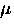SR

In aSR experiment, positively charged muons are implanted one at a time into the bulk of the superconductor. The muon is a lepton which has spin 1/2, a rest mass that is 206.729 times that of an electron, and a magnetic moment of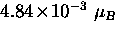.In the cuprates the muon comes to rest at a definite position in the crystallographic unit cell--becoming hydrogen bonded to an oxygen atom . Fortunately, in magnetic fields appropriate for aSR experiment, the intervortex spacing is much larger than the dimensions of the crystallographic unit cell (typically 102-103 times larger) so that a muon stops randomly on the length scale of the vortex lattice. The implanted muon precesses about the local magnetic field B with a Larmor frequency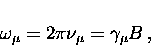(4)
where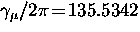MHz/T is the muon gyromagnetic ratio. After a mean lifetime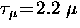s, the positive muon decays into a positron and two neutrinos (i.e.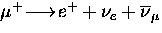). The distribution of decay positrons is asymmetric with respect to the spin polarization vector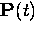of the muon, where the highest probability of emission is along the direction of the muon spin. Consequently, the time evolution of the muon spin polarizationcan be monitored, since the muon partially reveals its spin direction at the time of decay.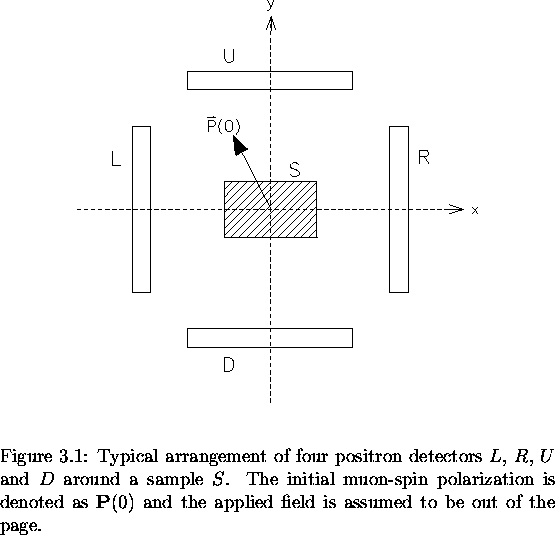Scintillation detectors placed around the sample can be used to detect the positrons emerging from the muon decay. Figure 3.1 shows a simple four-counter arrangement. The number of decay positrons recorded per time bin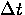in the ith counter is given by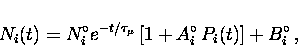(5)
where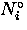is a normalization constant,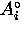is the maximum precession amplitude,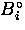is a time-independent random background and Pi(t) is the time evolution of the muon spin polarization component in the ith direction, which is given by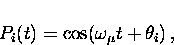(6)
where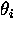is the initial phase of the muon spin polarization vector relative to the ith direction. Generallyis nonzero, since the muons will precess during their flight through the magnetic field to the sample. There is no significant loss of polarization during the short time over which the muons thermalize. This is because the primary interactions by which the muons rapidly lose their initial kinetic energy are electrostatic in nature and hence do not affect the muon spin .Next: 3.2.1 The Raw Asymmetry Up: 3 The SR Technique Previous: 3.1 SR vs. NMR